# 掌控堆栈，确保系统稳定

### 1. 关于栈和堆的简介

#### 突破限制

``````int array;
array = 0x1234;``````

``````int* p = malloc(32 * sizeof(int));
p += 35;
*p = 0x1234;``````

#### 栈

• 局部变量
• 返回地址
• 函数参数
• 编译器临时变量
• 中断上下文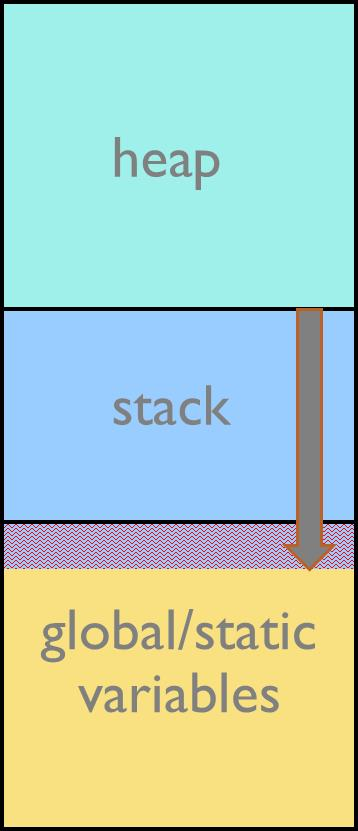### 2. 可靠的栈设计

#### 不同的设置栈大小的方法

``````char *highStack, *lowStack;
int main(int argc, char *argv[])
{
highStack = (char *)&argc;
// ...
printf("Current stack usage: %d\n", highStack - lowStack);
}``````
``````void deepest_stack_path_function(void)
{
int a;
lowStack = (char *)&a;
// ...
}``````

``````void sampling_timer_interrupt_handler(void)
{
char* currentStack;
int a;
currentStack = (char *)&a;
if (currentStack < lowStack) lowStack = currentStack;
}``````

#### 栈保护区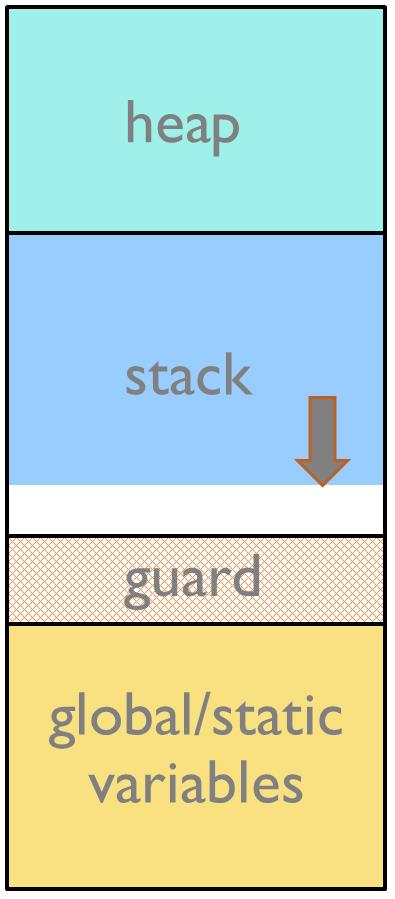#### 使用特定模式填充栈区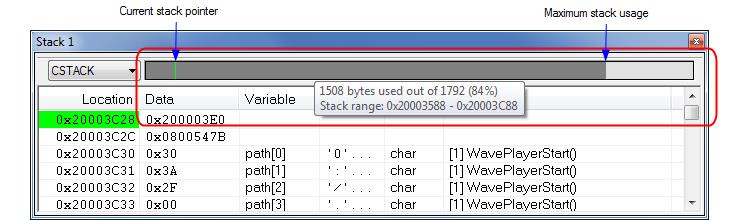#### 链接器计算的最大栈需求量

``````void
foo(int i)
{
#pragma calls = fun1, fun2, fun3
func_arr[i]();
}``````

• 至少有一个函数没有栈使用信息。
• 应用中至少有一个间接调用没有提供可能被调用的函数列表。
• 没有已知的间接调用，但至少有一个没有被调用的函数不是已知的根函数。
• 应用程序中出现递归（调用图中的循环）。
• 出现调用的函数被声明为根函数。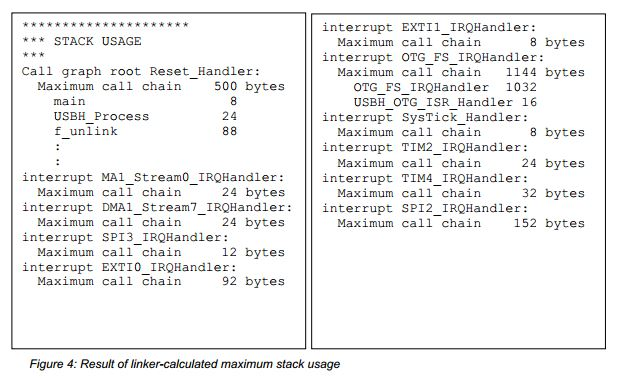### 3. 关于堆的简介

• 临时数据对象
• C++ new/delete
• C++ STL containers
• C++ exceptions

### 4. 可靠的堆设计

#### 会出现什么错误？

• 覆盖堆数据（变量和指针）
• 堆内部结构损毁

``void* malloc(size_t size);``
• 分配size大小的内存
• 返回一个指向所分配内存块的指针
• 内存块不清零
• 如果没有剩余的内存，则返回 NULL
``free (void* p);``
• 释放 p 指向的内存空间
• 要求 p 在之前调用 malloc()、calloc() 或 realloc() 时已经被返回
• 必须避免对同一个 p 多次调用 free(p)
``void* calloc(size_t nelem, size_t elsize);``
• 类似于 malloc()
• 清零内存块
``void* realloc(void* p, size_t size);``
• 类似于 malloc()
• 增加/缩减一个先前分配的块
• 返回的块可能有一个新的地址
``C++``
• new operator – 类似于malloc()
• new[]
• delete operator – 类似于 free()
• delete[]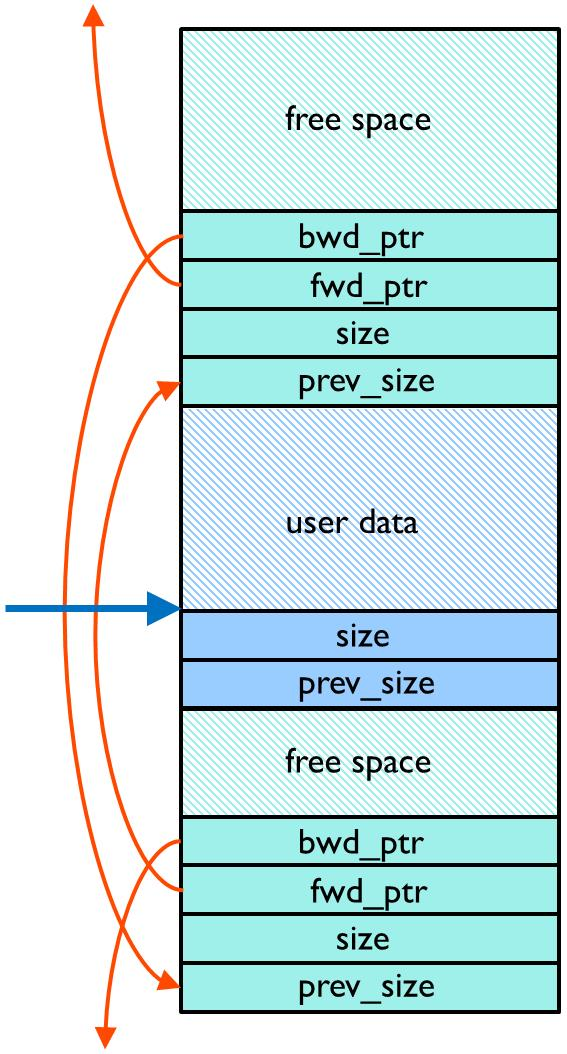#### 预防堆错误

• 初始化错误

• 错误地区分标量和数组

C++ 对标量和数组有不同的操作符，new 和 delete 用于标量，new[] 和 delete[] 用于数组。

• 向已被释放的内存写入数据

• 未检查返回值

malloc()、realloc() 和 calloc() 在内存不足时都会返回 NULL 指针。出现这种情况时，桌面系统会产生一个内存空间不足的错误，因此在开发时可以很容易地检测到。嵌入式系统可能会从 0 地址开始编码，并且可能在出现更细微的错误时仍然存在。如果您的 MCU 具有内存保护单元，您可以将其设置为当应用程序试图向闪存和其他只执行区域写入数据时产生一个内存保护错误。

• 多次释放同一内存

• 写入的数据超出了分配的区域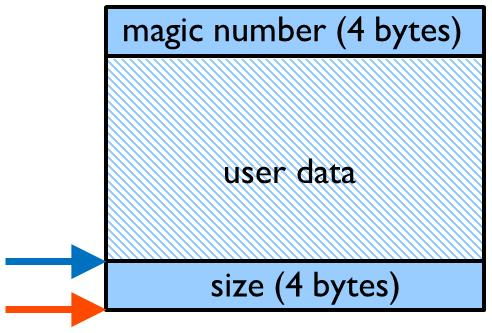``````#include <stdint.h>
#include <stdlib.h>
#define MAGIC_NUMBER 0xefdcba98
uint32_t myMallocMaxMem;

void* MyMalloc(size_t bytes)
{
uint8_t *p, *p_end;
static uint8_t* mLow = (uint8_t*)0xffffffff; /* lowest address
returned by
malloc() */
static uint8_t* mHigh; /* highest address + data returned by
malloc() */
bytes = (bytes + 3) & ~3; /* ensure alignment for magic number */
p = (uint8_t*)malloc(bytes + 8); /* add 2x32-bit for size and magic
number */
if (p == NULL)
{
abort(); /* out of memory */
}
*((uint32_t*)p) = bytes; /* remember size */
*((uint32_t*)(p + 4 + bytes)) = MAGIC_NUMBER; /* write magic number
after
user allocation */
/* crude method of estimating maximum used size since application
start */
if (p < mLow) mLow = p;
p_end = p + bytes + 8;
if (p_end > mHigh) mHigh = p_end;
myMallocMaxMem = mHigh - mLow;
return p + 4; /* allocated area starts after size */
}
void MyFree(void* vp)
{
uint8_t* p = (uint8_t*)vp - 4;
int bytes = *((uint32_t*)p);
/* check that magic number is not corrupted */
if (*((uint32_t*)(p + 4 + bytes)) != MAGIC_NUMBER)
{
abort(); /* error: data overflow or freeing already freed memory */
}
*((uint32_t*)(p + 4 + bytes)) = 0; /* remove magic number to be
able to
detect freeing already freed memory */
free(p);
}

#ifdef __cplusplus
// global override of operator new, delete, new[] and delete[]
void* operator new (size_t bytes) { return MyMalloc(bytes); }
void operator delete (void *p) { MyFree(p); }
#endif``````

### 结论

#### 参考资料：

1. Nigel Jones, blog posts at embeddedgurus.com, 2007 and 2009
2. John Regehr ,“Say no to stack overflow”, EE Times Design, 2004
3. Carnegie Mellon University , “Secure Coding in C and C++, Module 4, Dynamic Memory Management”, 2010

## 相关产品

We do no longer support Internet Explorer. To get the best experience of iar.com, we recommend upgrading to a modern browser such as Chrome or Edge.